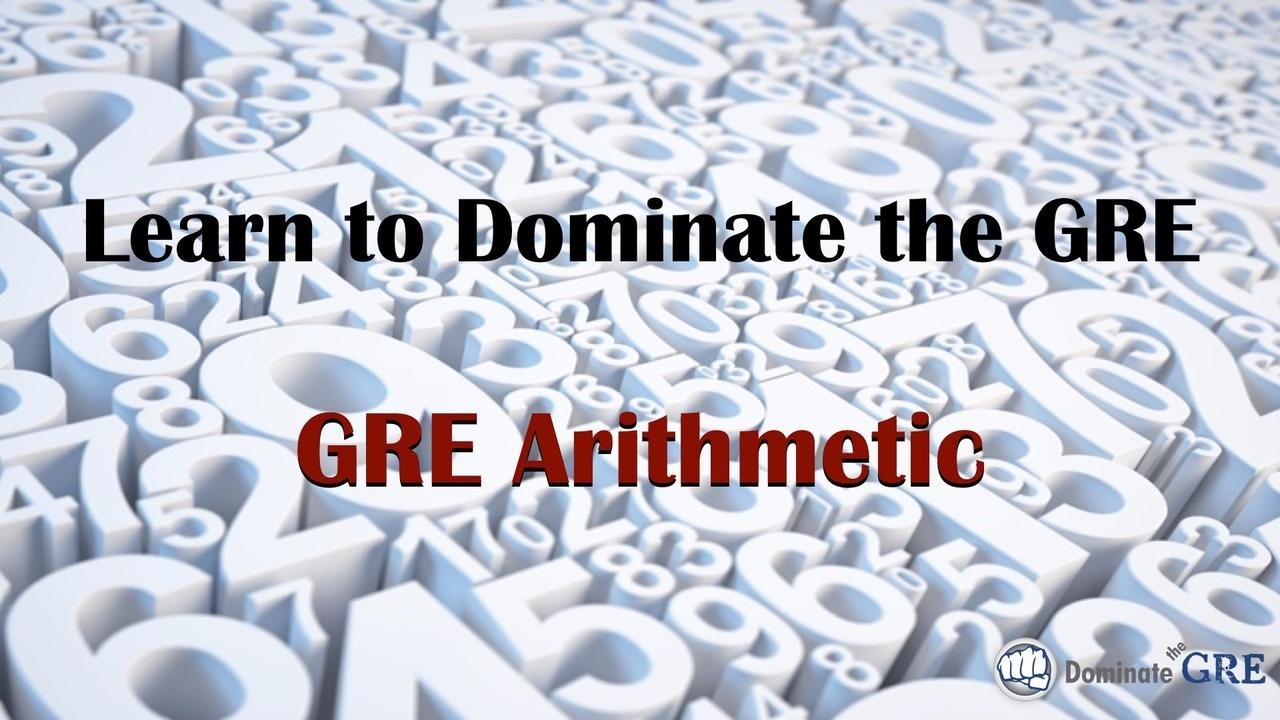# GRE Arithmetic - Video CourseSometimes GRE students just need to brush up on the basics. On the GRE quantitative section, approximately 1/3 of the questions will involve arithmetic in some way. When we say “arithmetic” we’re not talking about 2 + 2 = 4. Rather, we’re talking about questions that either directly or indirectly test mathematical concepts ranging from fractions to weighted averages to statistics to prime factorization. This video lesson covers all of the most important arithmetic concepts tested on the GRE with tricks, shortcuts, and direct application questions to help you not only dust off those “math cobwebs,” but also get more right answers on test day.

### Specifically, the GRE video tutorials in this module cover:

• Percents, fractions, and decimals with an emphasis on common GRE question types that test these concepts;
• Ratios;
• Direct vs. Inverse variation;
• Basic averages and Weighted Averages, including a unique strategy for quickly and easily solving weighted average problems;
• Units conversions;
• Mixtures and Average Rate questions;
• Prime factorization, Least Common Multiples (LCM), and Greatest Common Factors (GCF);
• Remainders;
• Statistics (mean, median, mode, range, standard deviation);
• Strategies and tricks — including the “Magic X” — for more quickly executing GRE arithmetic;
• Numerous application examples to fully illustrate all of the concepts taught in this course;
• And more!

Oh, and did we mention that this course includes a practice worksheet that will give you the opportunity to apply what you learn to real sample GRE arithmetic questions? Each question of the worksheet comes with a detailed video answer explanation showing you the most efficient way to solve it.

So what are you waiting for? Take your GRE preparation to the next level. Add this course to your library now and prepare to dominate the GRE!